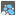• # Wrong algorithm,but almost works :)

## Question related to missionLoading Cargo

I do not have solved problem at this moment and I want to set aside it. But I have algorithm that crushed only on final test. Idea is wrong in root - we don't manage integers on 2 lists, but maybe it would be interesting for someone :)

```def checkio(data):
while len(data) > 1:
data.sort(reverse=True)
maxes_count = data.count(data)
if maxes_count == len(data):
data = data[2:]
else:
result = data[maxes_count - 1] - data[maxes_count]
del data[maxes_count]
data = result
return (data if len(data) == 1 else 0)

if __name__ == '__main__':
assert checkio([10, 10]) == 0, "1st example"
assert checkio() == 10, "2nd example"
assert checkio([5, 8, 13, 27, 14]) == 3, "3rd example"
assert checkio([5, 5, 6, 5]) == 1, "4th example"
assert checkio([12, 30, 30, 32, 42, 49]) == 9, "5th example"
assert checkio([1, 1, 1, 3]) == 0, "6th example"
```